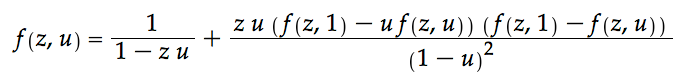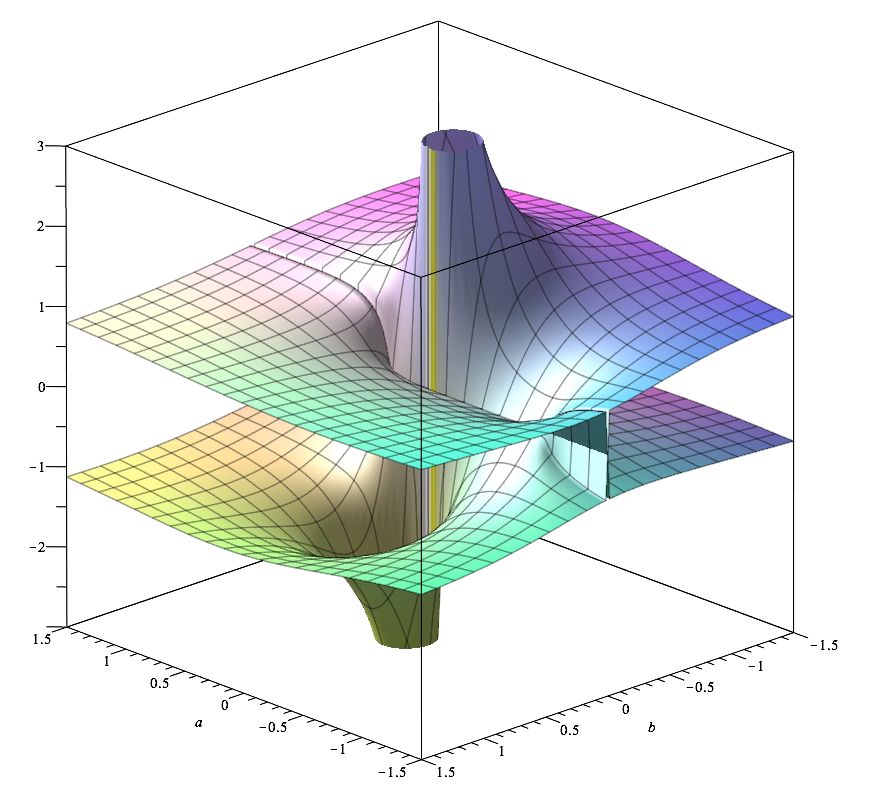# Jay Pantone

## Math 118

Winter 2016, Dartmouth College

This course starts by covering the symbolic method of Flajolet and Sedgewick, the asymptotic analysis of generating functions, and selected applications to combinatorial problems.

•  Lectures: M, W, F: 1:45pm - 2:50pm Haldeman 028
•  Office Hours: Monday, 4:00pm - 5:00pm Tuesday, 2:00pm - 3:00pm Thursday, 10:00am - 11:00am

##### Accompanying Code
• Topic 16: A collection of three python programs to simulate the distributions for binomial and Poisson random variables, as well as the distribution of the number of cycles in a random permutation.

• binomial.py – Simulates repeated trials of a binomial draw with n binary experiments, T at a time.
• python binomial.py n T &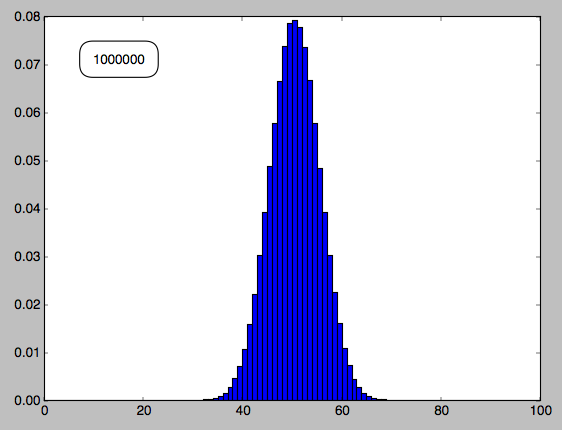• poisson.py – Simulates repeated trials of a Poisson draw with mean r, T at a time.
• python poisson.py r T &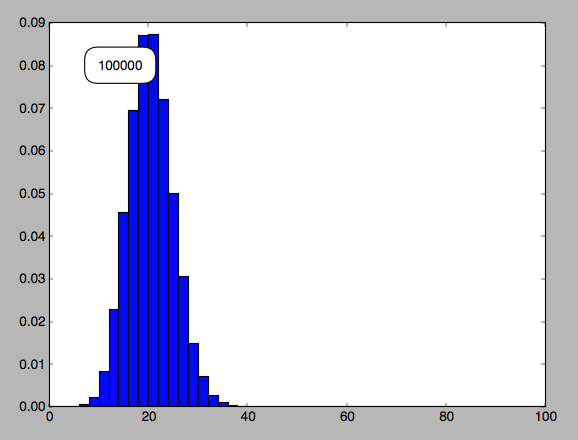• num-cycles-dist.py – Simulates the distribution of the number of cycles in a permutation of length n, T at a time.
• python num-cycles-dist.py n T &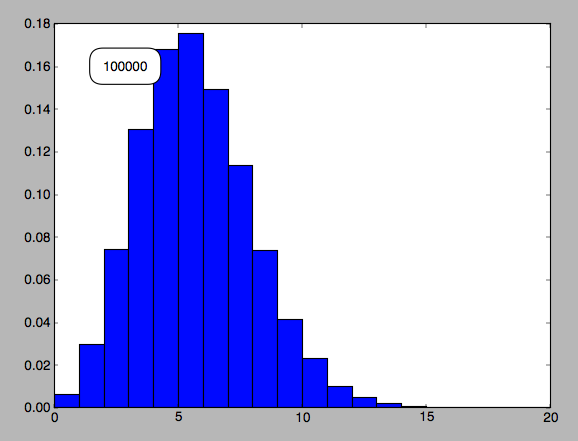• Topic 18: Maple Worksheet to compute the minimal polynomial for 2-West-stack-sortable permutations